## Three-Dimensional Coordinate Systems

The three-dimensional coordinate system expresses a point in space with three parameters, often length, width and depth ($x$, $y$, and $z$).

### Learning Objectives

Identify the number of parameters necessary to express a point in the three-dimensional coordinate system

### Key Takeaways

#### Key Points

• There are many types of coordinate systems, including Cartesian, spherical, and cylindrical coordinates.
• In the Cartesian system, all three of the parameters are represented as the quantitative distance from the reference plane.
• In order to convert from Cartesian to spherical, you need to convert each parameter separately, as follows: $r = \sqrt{x^2+y^2+z^2}\\ \theta=\arccos (\frac{z}{r})\\ \varphi =\arctan (\frac{y}{x})$

#### Key Terms

• coordinate system: a method of representing points in a space of given dimensions by coordinates from an origin
• origin: the point at which the axes of a coordinate system intersect

### The Three Dimensional Coordinate System

A three dimensional space has three geometric parameters: $x$, $y$, and $z$. These are often referred to as length, width and depth. Each parameter is perpendicular to the other two, and cannot lie in the same plane. shows a Cartesian coordinate system that uses the parameters $x$, $y$, and $z$.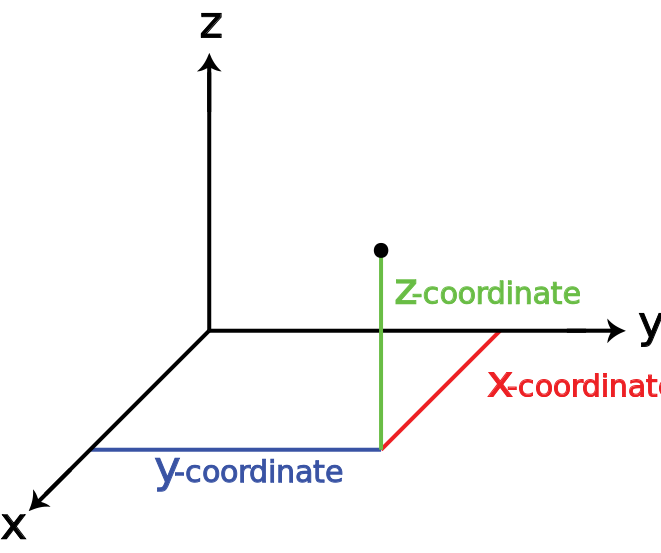Three-Dimensional Space: This is a three dimensional space represented by a Cartesian coordinate system.

### Cartesian Geometry

Also known as analytical geometry, this system is used to describe every point in three dimensional space in three parameters, each perpendicular to the other two at the origin. Each parameter is labeled relative to its axis with a quantitative representation of its distance from its plane of reference, which is determined by the other two parameter axes.

### Cylindrical Coordinates ($\rho, \varphi, z$)

The cylindrical system uses two linear parameters and one radial parameter:

• $\rho$: the radial distance from the point to $z$
• $\varphi$: the angle between the reference direction and the point
• $z$: the distance from the reference plane to the point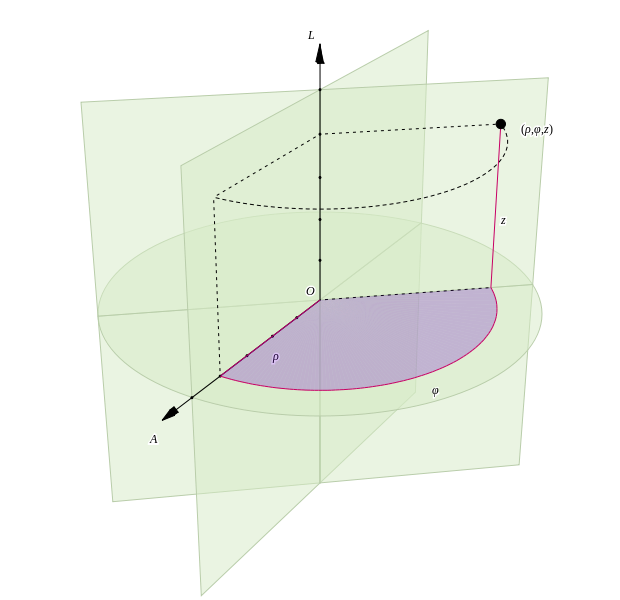Cylindrical Coordinate System: The cylindrical coordinate system is like a mix between the spherical and Cartesian system, incorporating linear and radial parameters.

### Spherical Coordinates ($r$, $\theta$, $\varphi$)

The spherical system is used commonly in mathematics and physics:

• $r$: the radial distance from the origin to the point
• $\theta$: the angle between the zenith direction and directional vector of $r$
• $\varphi$: the angle from the reference direction to the orthogonal plane projected by the directional vector of $r$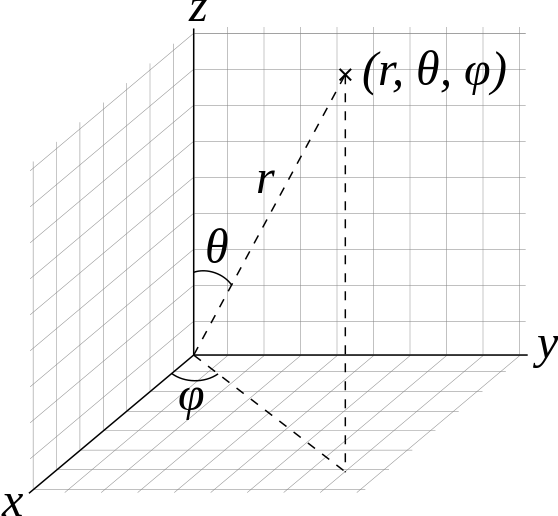Spherical Coordinate System: The spherical system is used commonly in mathematics and physics and has variables of $r$, $\theta$, and $\varphi$.

### Cartesian to Spherical

Often, you will need to be able to convert from spherical to Cartesian, or the other way around. The following equations will allow you to do just that:

$\displaystyle{r = \sqrt{x^2+y^2+z^2}}$

$\displaystyle{\theta=\arccos \left(\frac{z}{r}\right)}$

$\displaystyle{\varphi =\arctan \left(\frac{y}{x}\right)}$

## Vectors in the Plane

Vectors are needed in order to describe a plane and can give the direction of all dimensions in one vector equation.

### Learning Objectives

Calculate the directions of the normal vector and the directional vector of a reference point

### Key Takeaways

#### Key Points

• In order to adequately describe a plane, you need more than a point—you need a normal vector.
• The normal vector is perpendicular to the directional vector of the reference point.
• You can find the equation of a vector that describes a plane by using the following equation: $a (x-x_0) + b ( y-y_0) + c(z-z_0)=0$.

#### Key Terms

• vector: a directed quantity, one with both magnitude and direction; the signed difference between two points
• normal: a line or vector that is perpendicular to another line, surface, or plane

### Vectors in the Plane

Planes in a three dimensional space can be described mathematically using a point in the plane and a vector to indicate its “inclination”.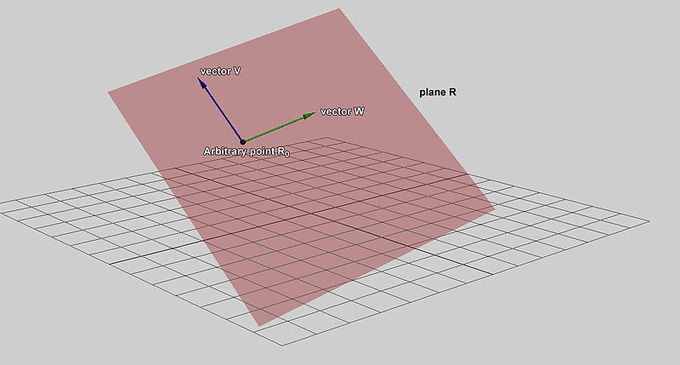Normal Vector to a Plane: This plane may be described parametrically as the set of all points of the form$\mathbf R = \mathbf {R}_0 + s \mathbf{V} + t \mathbf{W}$, where $s$ and $t$ range over all real numbers, $\mathbf{V}$ and $\mathbf{W}$ are given linearly independent vectors defining the plane, and $\mathbf{R_0}$ is the vector representing the position of an arbitrary (but fixed) point on the plane. The vectors $\mathbf{V}$ and $\mathbf{W}$ can be visualized as vectors starting at $\mathbf{R_0}$ and pointing in different directions along the plane. Note that $\mathbf{V}$ and $\mathbf{W}$ can be perpendicular but not parallel.

### General form of the equation of the plane

In order to find the equation of the plane, consider the following: Let $\mathbf{r}_0$ be the position vector of some point $\mathbf{P_0} = (x_0,y_0,z_0)$, and let $\mathbf{n} = (a,b,c)$ be a nonzero vector.

The plane determined by this point and vector consists of those points $P$, with position vector $\mathbf{r}$, such that the vector drawn from $P_0$ to $P$ is perpendicular to $\mathbf{n}$. Recall that two vectors are perpendicular if and only if their dot product is zero. As such, the equation that describes the plane is given by:

$\mathbf{n} \cdot (\mathbf{r}-\mathbf{r}_0)=0$

We can expand this equation in terms of its components to give:

$a (x-x_0)+ b(y-y_0)+ c(z-z_0)=0$

which we call the point-normal equation of the plane and is the general equation we use to describe the plane.

## Vectors in Three Dimensions

A Euclidean vector is a geometric object that has magnitude (i.e. length) and direction.

### Learning Objectives

Practice representing an Euclidean vector in the Cartesian coordinate system

### Key Takeaways

#### Key Points

• Vectors play an important role in physics.
• In the Cartesian coordinate system, a vector can be represented by identifying the coordinates of its initial and terminal point.
• The coordinate representation of vectors allows the algebraic features of vectors to be expressed in a convenient numerical fashion.
• Vectors can be added to other vectors according to vector algebra.

#### Key Terms

• tensor: a multidimensional array satisfying a certain mathematical transformation
• pseudovector: a quantity that transforms like a vector under a proper rotation but gains an additional change of sign under an improper rotation

A Euclidean vector (sometimes called a geometric or spatial vector, or—as here—simply a vector) is a geometric object that has magnitude (or length) and direction and can be added to other vectors according to vector algebra. A Euclidean vector is frequently represented by a line segment with a definite direction, or graphically as an arrow, connecting an initial point $A$ with a terminal point $B$, and denoted by $\vec{AB}$.

Vectors play an important role in physics: velocity and acceleration of a moving object and forces acting on it are all described by vectors. Many other physical quantities can be usefully thought of as vectors. The mathematical representation of a physical vector depends on the coordinate system used to describe it. Other vector-like objects that describe physical quantities and transform in a similar way under changes of the coordinate system include pseudovectors and tensors.

In the Cartesian coordinate system, a vector can be represented by identifying the coordinates of its initial and terminal point. For instance, in three dimensions, the points $A=(1,0,0)$ and $B=(0,1,0)$ in space determine the free vector $\vec{AB}$ pointing from the point $x=1$ on the $x$-axis to the point $y=1$ on the $y$-axis.

Typically in Cartesian coordinates, one considers primarily bound vectors. A bound vector is determined by the coordinates of the terminal point, its initial point always having the coordinates of the origin $O = (0,0,0)$. Thus the bound vector represented by $(1,0,0)$ is a vector of unit length pointing from the origin along the positive $x$-axis. The coordinate representation of vectors allows the algebraic features of vectors to be expressed in a convenient numerical fashion. For example, the sum of the vectors $(1,2,3)$ and $(−2,0,4)$ is the vector:

\begin{align} (1, 2, 3) + (-2, 0, 4) &= (1 -2, 2 + 0, 3 + 4) \\ &= (-1, 2, 7) \end{align}.Vector in 3D Space: A vector in the 3D Cartesian space, showing the position of a point $A$ represented by a black arrow. $\mathbf{i}$, $\mathbf{j}$, and $\mathbf{k}$ are unit vectors along the $x$-, $y$-, and $z$-axes, respectively.

## The Dot Product

The dot product takes two vectors of the same dimension and returns a single value.

### Learning Objectives

Formulate properties of the dot product, including the algebraic and geometric methods used to calculate it

### Key Takeaways

#### Key Points

• The dot product can be found algebraically or geometrically. The algebraic method employs the sum of the products of corresponding parameters, and the geometric method uses the product of the magnitudes of the vectors and the cosine of the angle between them.
• The dot product is a commutative property.
• The dot product is a distributive property.

#### Key Terms

• commutative: such that the order in which the operands are taken does not affect their image under the operation
• cross product: also called a vector product; results in a vector which is perpendicular to both of the vectors being multiplied and therefore normal to the plane containing them

### The Dot Product

The dot product takes two vectors and returns a single value. The dot product can only be taken from two vectors of the same dimension. The dot product is the sum of the product of the corresponding parameters. Geometrically, the dot product is the product of the magnitudes of two vectors and the cosine of the angle between them. This is different from the cross product, which gives an answer in vector form.Dot Product: When finding the dot product geometrically, you need the magnitudes of the vectors and the angle between them.

There are two representations of the dot product:

1. $\vec a \cdot \vec b = a_1b_1 + a_2b_2 + a_3b_3$
2. $\vec A \cdot \vec B = | A | |B | \cos \theta$

### Properties

Some of the properties of the dot product are

• The dot product is a commutative property, which means that the order of the terms does not change the outcome: $\vec a \cdot \vec b = \vec b \cdot \vec a$
• The dot product is a distributive property: $\vec a \cdot ( \vec b+ \vec c ) = \vec a \cdot \vec b + \vec a \cdot \vec c$
• If two vectors are normal (perpendicular) to each other, their dot product will be equal to zero: $\vec a \cdot \vec b = 0$

### Example

Find the dot product of the two vectors $\vec{Q}(5,2,8)$ and $\vec{R}(6,-2,9)$:

$\vec Q \cdot \vec R = Q_1 R_1 + Q_2 R_2 + Q_3 R_3 \\ = 5 \cdot 6 + 2 \cdot ( -2) + 8 \cdot 9 \\ = 98$

## The Cross Product

The cross product of two vectors is a vector which is perpendicular to both of the original vectors.

### Learning Objectives

Calculate the cross product of two vectors

### Key Takeaways

#### Key Points

• Since the cross product is perpendicular to both original vectors, it is also normal to the plane of the original vectors.
• If the two original vectors are parallel to each other, the cross product is zero.
• The cross product can be found both algebraically and geometrically.

#### Key Terms

• parallelogram: a convex quadrilateral in which each pair of opposite edges is parallel and of equal length
• perpendicular: at or forming a right angle (to)

The cross product is a binary operation of two three-dimensional vectors. The result is a vector which is perpendicular to both of the original vectors. Because it is perpendicular to both original vectors, the resulting vector is normal to the plane of the original vectors.

If the two original vectors are parallel to each other, the cross product will be zero.

The cross product is denoted as $a \times b = c$.

The direction of vector $c$ can be found by using the right hand rule.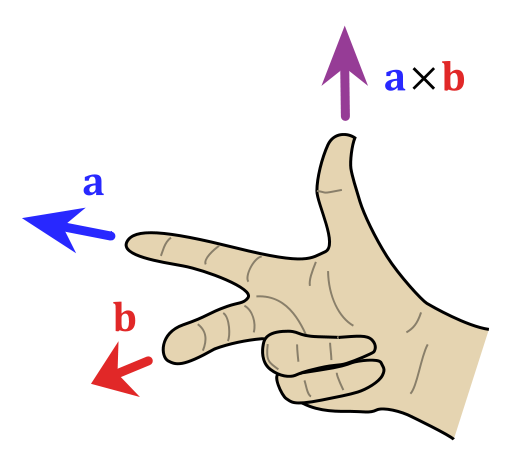The Right Hand Rule: If you use the rules shown in the figure, your thumb will be pointing in the direction of vector $c$, the cross product of the vectors.

The magnitude of vector $c$ is equal to the area of the parallelogram made by the two original vectors.

The cross product is different from the dot product because the answer is in vector form in the same number of dimensions as the original two vectors, where the dot product is given in the form of a single quantity in one dimension.

The cross product can be found both algebraically and geometrically.

The geometric method of finding the cross product uses the magnitudes of the vectors and the sine of the angle between them:

$a \times b = \left| a \right| \left| b \right| \sin \theta$

The algebraic method of finding the cross product of two vectors involves inputting the vector information into matrices and manipulating them:

$=\left[ {\begin{array}{cc} a_2 & a_3 \\ b_2 & b_3 \\ \end{array} } \right]i - \left[ {\begin{array}{cc} a_1 & a_3 \\ b_1 & b_3 \\ \end{array} } \right]j + \left[ {\begin{array}{cc} a_1 & a_2 \\ b_1 & b_2 \\ \end{array} } \right]k\\ =\left[ {\begin{array}{cc} i & j & k \\ a_1 & a_2 & a_3 \\ b_1 & b_2 & b_3 \\ \end{array} } \right]\\$

The manipulated matrices form the following equations:

$=< a_2b_3 - a_3b_2, a_3b_1 - a_1b_3, a_1b_2 - a_2b_1 >$

## Equations of Lines and Planes

A line is a vector which connects two points on a plane and the direction and magnitude of a line determine the plane on which it lies.

### Learning Objectives

Explain the relationship between the lines and three dimensional geometric objects

### Key Takeaways

#### Key Points

• The slope of the line, and the plane it lies on, is the angle of inclination of that line.
• A line is a two dimensional representation of a three dimensional geometric object, a plane.
• The parametric equation of a line is written as: $<x, y, z> \ = \ <x_0 + at, y_0+bt, z_0 + ct>$

#### Key Terms

• vector: a directed quantity, one with both magnitude and direction; the signed difference between two points
• slope: also called gradient; slope or gradient of a line describes its steepness

### Lines and Planes

A line is described by a point on the line and its angle of inclination, or slope. Every line lies in a plane which is determined by both the direction and slope of the line. A line is essentially a representation of a cross section of a plane, or a two dimensional version of a plane which is a three dimensional object.

### Equations of Lines and Planes

The components of equations of lines and planes are as follows:

A line in three dimensional space is given by a point, $P_0 (x_o,y_o,z_o)$, or a plane, $M$, and its direction. This direction is described by a vector, $\mathbf{v}$, which is parallel to plane and $P$ is the arbitrary point on plane $M$.

The position vector of point $P_0$ is called $\mathbf{r}_0$ and the position vector of point $P$ is called $\mathbf{r}$. The vector from $P$ to $P_0$ is called vector $\mathbf{a}$. Vectors $\mathbf{a}$ and $\mathbf{v}$ are parallel to each other.

Now, we can use all this information to form the equation of a line on plane $M$.

The vector equation of a line is:

$\mathbf{r} = \mathbf{r}_0 + t \mathbf{v}$

where $t$ represents the location of vector $\mathbf{r}$ on plane $M$.

The parametric equation of a line can be written as:

$x = x_0 + at \\ y=y_0 +bt \\ z = z_0 +ct$

Or the more compact form:

$<x, y, z> \ = \ <x_0 + at, y_0+bt, z_0 + ct>$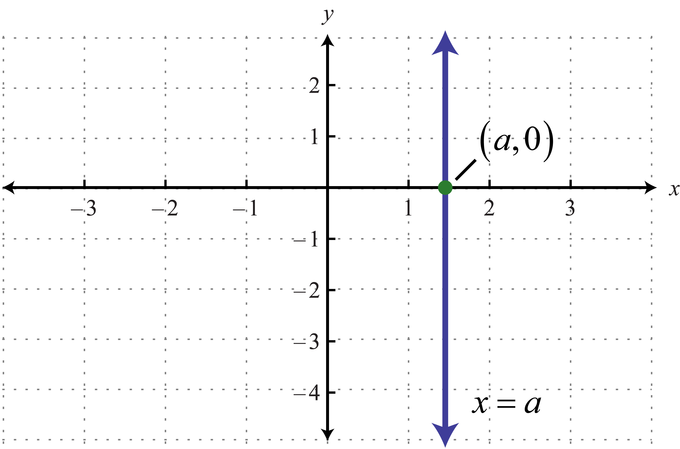Vertical Line, Graphed: Vertical line $x = a$, lying on the $xy$-plane ($z=0$).

A quadric surface is any $D$-dimensional hypersurface in $(D+1)$-dimensional space defined as the locus of zeros of a quadratic polynomial.

### Key Takeaways

#### Key Points

• A cylinder is one of the most basic curvilinear geometric shapes, the surface formed by the points at a fixed distance from a given line segment, the axis of the cylinder.
• A cylinder can be seen as a polyhedral limiting case of an n-gonal prism where n approaches infinity.
• A quadric, or quadric surface, is any $D$-dimensional hypersurface in $(D+1)$-dimensional space defined as the locus of zeros of a quadratic polynomial.
• Cylinders, spheres, ellipsoids, etc. are special cases of quadric surfaces.

#### Key Terms

• polynomial: an expression consisting of a sum of a finite number of terms, each term being the product of a constant coefficient and one or more variables raised to a non-negative integer power
• hypersurface: a $n$-dimensional surface in a space (often a Euclidean space) of dimension $n+1$

### Cylinder

A cylinder (from Greek “roller” or “tumbler”) is one of the most basic curvilinear geometric shapes. The surface is formed by the points at a fixed distance from a given line segment, the axis of the cylinder. The solid enclosed by this surface and by two planes perpendicular to the axis is also called a cylinder. The surface area and the volume of a cylinder have been known since antiquity. A cylinder can be seen as a polyhedral limiting case of an $n$-gonal prism where $n$ approaches infinity.Cylinder: A right circular cylinder with radius $r$ and height $h$.

In common use, a cylinder is taken to mean a finite section of a right circular cylinder, i.e. the cylinder with the generating lines perpendicular to the bases, with its ends closed to form two circular surfaces. If the cylinder has a radius $r$ and length (height) $h$, then its volume is given by $V = \pi r^2h$, and its surface area is $A = 2\pi rh$ without the top and bottom, and $2\pi r(r + h)$ with them.

A quadric, or quadric surface, is any $D$-dimensional hypersurface in $(D+1)$-dimensional space defined as the locus of zeros of a quadratic polynomial. In coordinates $\{x_1, x_2, \cdots, x_{D+1} \}$, the general quadric is defined by the algebraic equation:

$\displaystyle{\sum_{i,j=1}^{D+1} x_i Q_{ij} x_j + \sum_{i=1}^{D+1} P_i x_i + R = 0}$

Cylinders, spheres, ellipsoids, etc. are special cases of quadric surfaces.

### Examples

Ellipsoid:

$\displaystyle{{x^2 \over a^2} + {y^2 \over b^2} + {z^2 \over c^2} = 1}$

Sphere:

$\displaystyle{{x^2 \over a^2} + {y^2 \over a^2} + {z^2 \over a^2} = 1}$

Elliptic paraboloid:

$\displaystyle{{x^2 \over a^2} + {y^2 \over b^2} - z = 0}$

Cone:

$\displaystyle{{x^2 \over a^2} + {y^2 \over b^2} - {z^2 \over c^2} = 0}$

Parabolic cylinder:

$x^2 + 2ay = 0$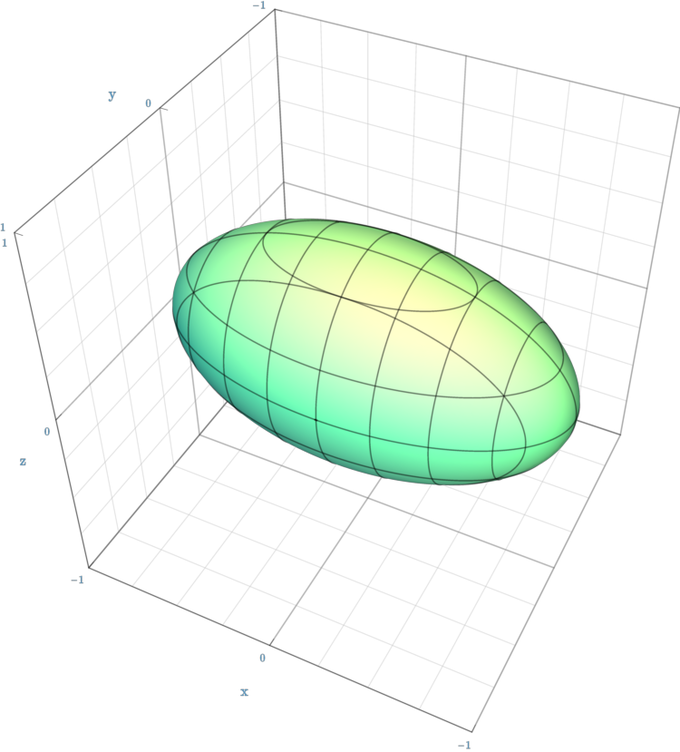Ellipsoid: An ellipsoid given as ${x^2 \over a^2} + {y^2 \over b^2} + {z^2 \over c^2} = 1$.

## Cylindrical and Spherical Coordinates

Cylindrical and spherical coordinates are useful when describing objects or phenomena with specific symmetries.

### Learning Objectives

Distinguish between cylindrical and spherical coordinate systems

### Key Takeaways

#### Key Points

• A cylindrical coordinate system is a three-dimensional coordinate system that specifies point positions by three numbers $(\rho, \varphi, z)$.
• Cylindrical coordinates are useful in connection with objects and phenomena that have some rotational symmetry about the longitudinal axis.
• A spherical coordinate system is a coordinate system for three-dimensional space where the position of a point is specified by three numbers $(r, \theta, \varphi)$.

#### Key Terms

• azimuth: an arc of the horizon intercepted between the meridian of the place and a vertical circle passing through the center of any object
• Cartesian: of or pertaining to co-ordinates based on mutually orthogonal axes

While Cartesian coordinates have many applications, cylindrical and spherical coordinates are useful when describing objects or phenomena with specific symmetries.

### Cylindrical Coordinates

A cylindrical coordinate system is a three-dimensional coordinate system that specifies point positions by the distance from a chosen reference axis, the direction from the axis relative to a chosen reference direction, and the distance from a chosen reference plane perpendicular to the axis. The latter distance is given as a positive or negative number, depending on which side of the reference plane faces the point.Cylindrical Coordinate System: A cylindrical coordinate system with origin $O$, polar axis $A$, and longitudinal axis $L$. The dot is the point with radial distance $\rho = 4$, angular coordinate $\varphi = 130$ degrees, and height $z = 4$.

Cylindrical coordinates are useful in connection with objects and phenomena that have some rotational symmetry about the longitudinal axis, such as water flow in a straight pipe with a round cross-section, heat distribution in a metal cylinder, electromagnetic fields produced by an electric current in a long, straight wire, and so on.

For the conversion between cylindrical and Cartesian coordinate co-ordinates, it is convenient to assume that the reference plane of the former is the Cartesian $xy$-plane (with equation $z = 0$), and the cylindrical axis is the Cartesian $z$-axis. Then the $z$ coordinate is the same in both systems, and the correspondence between cylindrical $(\rho,\varphi)$ and Cartesian $(x,y)$ are the same as for polar coordinates, namely $x = \rho \cos \varphi; \, y = \rho \sin \varphi$. In the reverse transformation:

$\rho = \sqrt{x^{2}+y^{2}}$

$\varphi = \begin{cases} 0 & \mbox{if } x = 0 \mbox{ and } y = 0\\ \arcsin(\frac{y}{\rho}) & \mbox{if } x \geq 0 \\ -\arcsin(\frac{y}{\rho}) + \pi & \mbox{if } x < 0\\ \end{cases}$

### Spherical Coordinates

A spherical coordinate system is a coordinate system for three-dimensional space where the position of a point is specified by three numbers: the radial distance of that point from a fixed origin, its polar angle measured from a fixed zenith direction, and the azimuth angle of its orthogonal projection on a reference plane that passes through the origin and is orthogonal to the zenith, measured from a fixed reference direction on that plane. Spherical coordinates are useful in connection with objects and phenomena that have spherical symmetry, such as an electric charge located at the origin.Spherical Coordinate System: Spherical coordinates ($r$, $\theta$, $\varphi$) as often used in mathematics: radial distance $r$, azimuthal angle $\theta$, and polar angle $\varphi$. The meanings of $\theta$ and $\varphi$ have been swapped compared to the physics convention.

The spherical coordinates (radius $r$, inclination $\theta$, azimuth $\varphi$) of a point can be obtained from its Cartesian coordinates ($x$, $y$, $z$) by the formulae:

$r=\sqrt{x^2+y^2+z^2} \\ \theta = \mbox{arccos}\left(\frac{z}{r}\right) \\ \varphi = \mbox{arctan}\left(\frac{y}{x}\right)$

In reverse:

$x=r \, \sin\theta \, \cos\varphi \\ y=r \, \sin\theta \, \sin\varphi \\ z=r \, \cos\theta$

## Surfaces in Space

A surface is a two-dimensional, topological manifold.

### Learning Objectives

Explain the meaning of the two-dimensionality of surfaces

### Key Takeaways

#### Key Points

• To say that a surface is “two-dimensional” means that, about each point, there is a coordinate patch on which a two-dimensional coordinate system is defined.
• The concept of surface finds application in physics, engineering, computer graphics, and many other disciplines, primarily in representing the surfaces of physical objects.
• Surfaces could be the locus of zeros of certain functions, usually polynomial functions.

#### Key Terms

• parametric: of, relating to, or defined using parameters
• meridian: an imaginary great circle on the Earth’s surface, passing through the geographic poles
• manifold: a topological space that looks locally like the “ordinary” Euclidean space and is Hausdorff

A surface is a two-dimensional, topological manifold. The most familiar examples are those that arise as the boundaries of solid objects in ordinary three-dimensional Euclidean space $R^3$— for example, the surface of a ball. On the other hand, there are surfaces, such as the Klein bottle, that cannot be embedded in three-dimensional Euclidean space without introducing singularities or self-intersections.

To say that a surface is “two-dimensional” means that, about each point, there is a coordinate patch on which a two-dimensional coordinate system is defined. For example, the surface of the Earth is (ideally) a two-dimensional surface, and latitude and longitude provide two-dimensional coordinates on it (except at the poles and along the 180th meridian).

The concept of surface finds application in physics, engineering, computer graphics, and many other disciplines, primarily in representing the surfaces of physical objects. For example, in analyzing the aerodynamic properties of an airplane, the central consideration is the flow of air along its surface.

Historically, surfaces were initially defined as subspaces of Euclidean spaces. Often, these surfaces were the locus of zeros of certain functions, usually polynomial functions. Such a definition considered the surface as part of a larger (Euclidean) space, and as such was termed extrinsic.

### Example

In Cartesian coordinates, a sphere can be defined parametrically—e.g., by:

$x = r \sin \theta \cos \varphi\\ y=r\sin\theta\sin\varphi\\ z=r\cos\theta$

or implicitly—e.g., by:

$x^2 + y^2 + z^2 − r^2 = 0$

In spherical coordinates, the surface can be expressed simply by $r=R$.A Sphere Defined Parametrically: A sphere can be defined parametrically or implicitly.